Courses

# Critical Thickness Of Insulation And Heat Transfer From Extended Surfaces (Fins) - MCQ Test 1

## 15 Questions MCQ Test Mock Test Series - Mechanical Engineering (ME) for GATE 2020 | Critical Thickness Of Insulation And Heat Transfer From Extended Surfaces (Fins) - MCQ Test 1

Description
This mock test of Critical Thickness Of Insulation And Heat Transfer From Extended Surfaces (Fins) - MCQ Test 1 for Mechanical Engineering helps you for every Mechanical Engineering entrance exam. This contains 15 Multiple Choice Questions for Mechanical Engineering Critical Thickness Of Insulation And Heat Transfer From Extended Surfaces (Fins) - MCQ Test 1 (mcq) to study with solutions a complete question bank. The solved questions answers in this Critical Thickness Of Insulation And Heat Transfer From Extended Surfaces (Fins) - MCQ Test 1 quiz give you a good mix of easy questions and tough questions. Mechanical Engineering students definitely take this Critical Thickness Of Insulation And Heat Transfer From Extended Surfaces (Fins) - MCQ Test 1 exercise for a better result in the exam. You can find other Critical Thickness Of Insulation And Heat Transfer From Extended Surfaces (Fins) - MCQ Test 1 extra questions, long questions & short questions for Mechanical Engineering on EduRev as well by searching above.
QUESTION: 1

### A steel steam pipe 10 cm inner diameter and 11 cm outer diameter is covered with insulation having the thermal conductivity of 1 W/mK. If the convective heat transfer coefficient between the surface of insulation and the surrounding air is 8 W / m2K, then critical radius of insulation is:

Solution:

Ans. (c) Critical radius of insulation (rc) =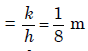= 12.5cm

QUESTION: 2

### For a current wire of 20 mm diameter exposed to air (h = 20 W/m2K),maximum heat dissipation occurs when thickness of insulation (k = 0.5W/mK) is:

Solution:

Ans. (b) Maximum heat dissipation occurs when thickness of insulation is critical.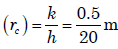= 25mm

Therefore thickness of insulation =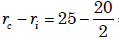= 15 mm

QUESTION: 3

### Upto the critical radius of insulation:

Solution:

Ans. (a)

QUESTION: 4

The value of thermal conductivity of thermal insulation applied to ahollow spherical vessel containing very hot material is 0·5 W/mK. The convective heat transfer coefficient at the outer surface of insulation is10 W/m2K.What is the critical radius of the sphere?

Solution:

Ans. (a) Minimum q at ro =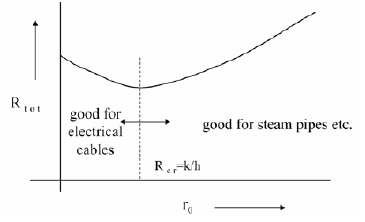QUESTION: 5

A metal rod of 2 cm diameter has a conductivity of 40W/mK, which is tobe insulated with an insulating material of conductivity of 0.1 W/m K. Ifthe convective heat transfer coefficient with the ambient atmosphere is5 W/m2K, the critical thickness of insulation will be:

Solution:

Ans. (a) Critical radius of insulation (rc) =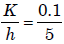= 0.02m = 2cm

Critical thickness of insulation (t) =rc - r1 = 2 - 1 = 1cm

QUESTION: 6

In order to substantially reduce leakage of heat from atmosphere intocold refrigerant flowing in small diameter copper tubes in a refrigerant system, the radial thickness of insulation, cylindrically wrapped around the tubes, must be:

Solution:

Ans. (d) At critical radius of insulation heat leakage is maximum if we add more
insulation then heat leakage will reduce.

QUESTION: 7

In a long cylindrical rod of radius R and a surface heat flux of qo the uniform internal heat generation rate is:

Solution:

Ans. (a)

QUESTION: 8

A plane wall of thickness 2L has a uniform volumetric heat source q* (W/m3). It is exposed to local ambient temperature T at both the ends (x = ± L). The surface temperature Ts of the wall under steady-state
condition (where h and k have their usual meanings) is given by:

Solution:

Ans. (a)

QUESTION: 9

A steam pipe is to be lined with two layers of insulating materials of different thermal conductivities. For minimum heat transfer

Solution:

Ans. (a) For minimum heat transfer, the better insulation must be put inside.

QUESTION: 10

An electric cable of aluminium conductor (k = 240 W/mK) is to be insulated with rubber (k = 0.15 W/mK). The cable is to be located in air (h = 6 W/m2). The critical thickness of insulation will be

Solution:
QUESTION: 11

Assertion (A): Addition of insulation to the inside surface of a pipealways reduces heat transfer rate and critical radius concept has nosignificance.
Reason (R): If insulation is added to the inside surface, both surfaceresistance and internal resistance increase.

Solution:

Ans. (a) A and R are correct. R is right reason for A.

QUESTION: 12

It is desired to increase the heat dissipation rate over the surface of anelectronic device of spherical shape of 5 mm radius exposed toconvection with h = 10 W/m2K by encasing it in a spherical sheath ofconductivity 0.04 W/mK, For maximum heat flow, the diameter of thesheath should be:

Solution:

Ans. (b) The critical radius of insulation for ensuring maximum heat transfer by
conduction (r) =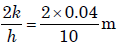= 8mm.

Therefore diameter should be 16 mm.

QUESTION: 13

From a metallic wall at 100°C, a metallic rod protrudes to the ambientair. The temperatures at the tip will be minimum when the rod is made of:

Solution:

Ans. (b)

QUESTION: 14

Usually fins are provided to increase the rate of heat transfer. But finsalso act as insulation. Which one of the following non-dimensional numbers decides this factor?

Solution:
QUESTION: 15

Which one of the following is correct?
Fins are used to increase the heat transfer from a surface by

Solution:

Ans. (b)